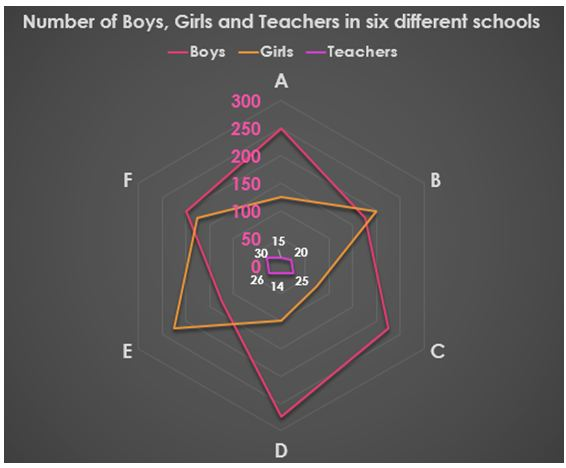# CWC/FCI Prelims 2019 – Quantitative Aptitude Questions (Day-37)

Dear Readers, Exam Race for the Year 2019 has already started, To enrich your preparation here we are providing new series of Practice Questions on Quantitative Aptitude – Section for CWC/FCI Exam. Aspirants, practice these questions on a regular basis to improve your score in aptitude section. Start your effective preparation from the right beginning to get success in upcoming CWC/FCI Exam.

[WpProQuiz 5867]

Directions (1 – 5): Study the following information carefully and answer the questions given below:1) Sum of total number of boys, girls and teachers in school A is what percent of the sum of total number of boys, girls and teachers in school D?

a) 101.23%

b) 100.25%

c) 102.32%

d) 103.15%

e) None of these

2) Find the respective ratio between total number of boys, girls and teachers in school B and that in school C.

a) 67:81

b) 81:67

c) 65:79

d) 79:65

e) None of these

3) Find the difference between total number of boys and total number of girls from all the schools together.

a) 300

b) 350

c) 450

d) 500

e) None of these

4) Total number of boys, girls and teachers of school E is what percent more/less than the total number of boys, girls and teachers of school F?

a) 7.1% less

b) 8.2% more

c) 7.1% more

d) 8.2% less

e) None of these

5) Find the sum of total number of teachers from all the schools together and total number of students of schools A and C.

a) 850

b) 660

c) 665

d) 805

e) None of these

Directions (6 – 10): In each of these questions two equations (I) and (II) are given. You have to solve both the equations and give answer

a) If x > y

b) If x ≥ y

c) If x < y

d) If x ≤ y

e) If x = y or no relation can be established between x and y

6)

I. 6x2 – 19x + 15 =0

II. 10y2 – 29y – 21 =0

7)

I. 5x2 -16x +11 =0

II. 5y2 -3y =2

8)

I. x2 + 18x+72 =0

II. Y2 + 6y +8 =0

9)

I. 8x2 -22x +15 =0

II. 3y2-13y +14 =0

10)

I. 9x2 -26x +16 =0

II. 3y2 -16y+ 20 =0

Directions (1-5):

Sum of total number of boys, girls and teachers in school A = 250 + 125 + 15

= 390

Sum of total number of boys, girls and teachers in school D = 275 + 100 + 14

= 389

Required percentage = 390/389 x 100 = 100.25%

Total number of boys, girls and teachers in school B = 175 + 200 + 20 = 395

Total number of boys, girls and teachers in school C = 225 + 75 + 25 = 325

Required ratio = 395:325 = 79:65

Total number of boys from all the schools together = 250 + 175 + 225 + 275 + 125 + 200 = 1250

Total number of girls from all the schools together = 125 + 200 + 75 + 100 + 225 + 175 = 900

Required difference = 1250 – 900 = 350

Total number of boys, girls and teachers of school E = 125 + 225 + 26 = 376

Total number of boys, girls and teachers of school F = 200 + 175 + 30 = 405

Required percentage = (405 – 376)/405 x 100 = 7.1% less

Total number of teachers from all the schools together

= 15 + 20 + 25 + 14 + 26 + 30 = 130

Total number of students of school A = 250 + 125 = 375

Total number of students of school C = 225 + 75 = 300

Required sum = 130 + 375 + 300 = 805

Directions (6-10):

I. 6x2 – 19x + 15 =0

6x2 – 9x-10x + 15 =0

3x(2x-3) -5(2x-3) =0

(3x-5) (2x-3) =0

x= 5/3, 3/2

II. 10y2 – 29y – 21 =0

10y2 – 35y +6y -21 =0

5y (2y-7)+3 (2y-7) =0

(5y+3) (2y-7) =0

y= -3/5, 7/2

Can’t be determined

I. 5x2 -16x +11 =0

5x2 -11x-5x +11 =0

x(5x-11)-1(5x-11)=0

(x-1) (5x-11)=0

x=1, 11/5

II. 5y2 – 3y – 2 =0

5y2 – 5y+2y – 2 =0

5y(y-1)+2(y-1)=0

(5y+2)(y-1) =0

y = -2/5, 1

Hence, x ≥ y

I. x2 + 18x+72 =0

x2 + 12x +6x +72 =0

x(x+12)+6 (x+12) =0

(x+6) (x+12) =0

x=-6, -12

II. Y2 + 6y +8 =0

Y2 + 4y+2y +8 =0

Y(y+4)+2 (y+4) =0

(y+2) (y+4) =0

Y =-2, -4

Hence, x<y

I. 8x2 -22x +15 =0

8x2 -12x-10x +15 =0

4x (2x-3)-5(2x-3) =0

(4x-5) (2x-3) =0

x= 5/4, 3/2

II. 3y2-13y +14 =0

3y2-6y -7y +14 =0

3y(y-2)-7(y-2) =0

(3y-7)(y-2)=0

y=7/3, 2

Hence, x<y

I. 9x2 -26x +16 =0

9x2 -18x -8x +16 =0

9x(x-2)-8 (x-2) =0

(9x-8) (x-2) =0

x=8/9, 2

II. 3y2 -16y+ 20 =0

3y2 -10y-6y + 20 =0

y (3y-10)-2(3y-10) =0

(y-2) (3y-10) =0

y =2, 10/3

Hence, x ≤ y